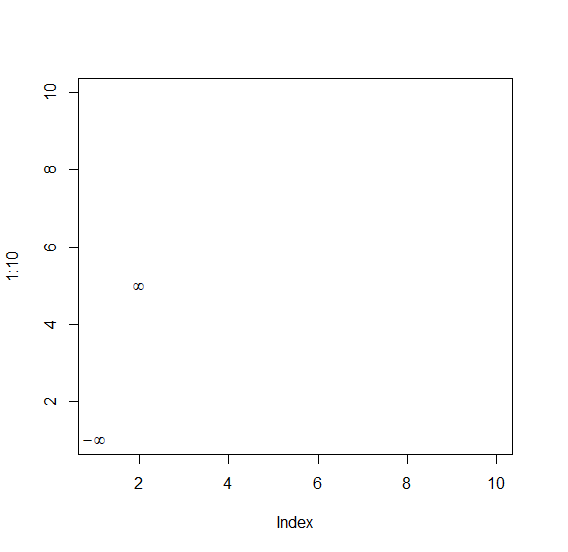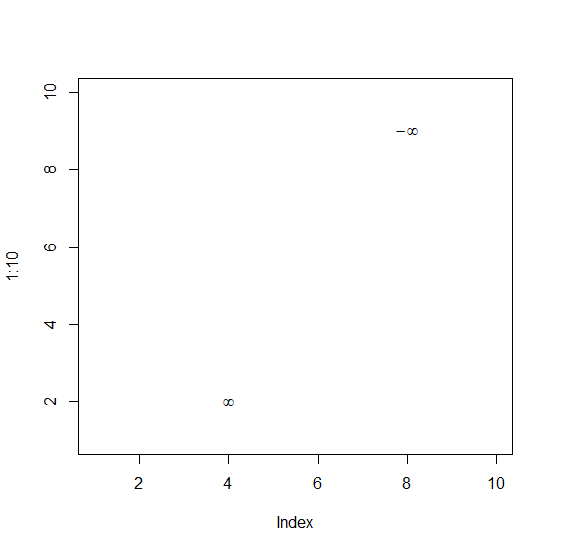# Display infinity and minus infinity both symbols in one base R plot.

R ProgrammingServer Side ProgrammingProgramming

To display infinity and minus infinity symbol in one base R plot, we can use text function and expression function. Inside expression function we can put infinity word for the display of infinity and infinity word with minus sign for the display of minus infinity.

Check out the below Examples to understand how it can be done.

## Example 1

To display infinity and minus infinity symbol in one base R plot use the following code −

plot(1:10,type="n")
text(c(1,5),expression(-infinity,infinity))

## Output

If you execute the above given snippet, it generates the following Output −## Example 2

To display infinity and minus infinity symbol in one base R plot use the following code −

plot(1:10,type="n")
text(c(4,8),c(2,9),expression(infinity,-infinity))

## Output

If you execute the above given snippet, it generates the following Output −